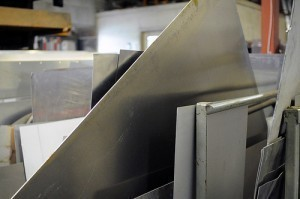•• # Aluminum Sheet Gauge Sizes

Aluminum sheet gauge sizes are used to determine the thickness of a metal sheet piece. The thinner the sheet is, the higher the number will be.

### Gauge Thickness GuideGauge 000000 is 0.5800 inch thick. Gauge 0000 is 0.4600 thick, while 00 is 0.3648 inch. Gauge 1, 2, 3 and 4 have thicknesses of 0.2893, 0.2576, 0.2294 and 0.2043 inches. Gauge sizes 5, 6, 7 and 8 are 0.1819, 0.1620, 0.1443 and 0.1285 inches thick respectively. Gauge 12 is 0.1046 thick, while gauge 14 is 0.0747 inch thick. Gauge 16 is 0.0598, while gauge 18 has a thickness of 0.0478 of an inch.

### Guide for Aluminum Gauge Measurement

You will need a caliper and a gauge conversion list. Open the caliper and make it wider than the aluminum metal. Close the caliper jaws on the sheet. Position the jaws and set their edges on the aluminum.

Write the thickness down. Repeat these steps for all the metal edges. Combine the values. To get the thickness, divide the sum in four. The result is the average thickness. Use a conversion sheet and find the thickness nearest the figure you got. Find the matching gauge number.